# NCERT Solutions for Class 9 Maths chapter 12 Exercise 12.2 - Heron’s Formula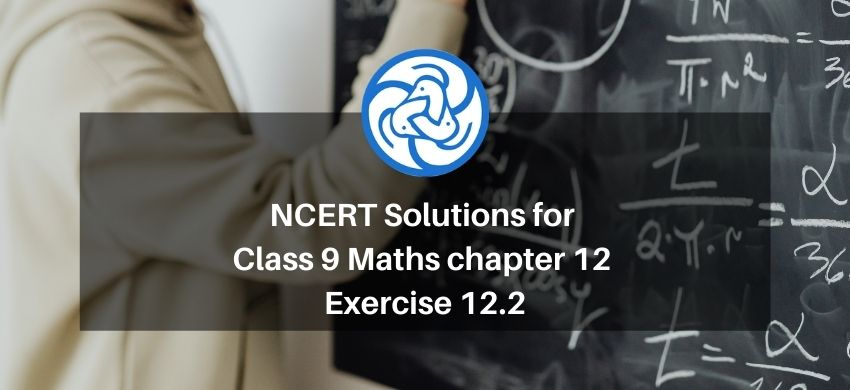Hey, are you a class 9 Student and Looking for Ways to Download NCERT Solutions for Class 9 Maths chapter 12 Exercise 12.2? If Yes then you are at the right place.

Here we have listed Class 9 maths chapter 12 exercise 12.2 solutions in PDF that is prepared by Kota’s top IITian’s Faculties by keeping Simplicity in mind.

If you want to score high in your class 9 Maths Exam then it is very important for you to have a good knowledge of all the important topics, so to learn and practice those topics you can use eSaral NCERT Solutions.

So, without wasting more time Let’s start.

### Download The PDF of NCERT Solutions for Class 9 Maths chapter 12 Exercise 12.2 "Heron’s Formula"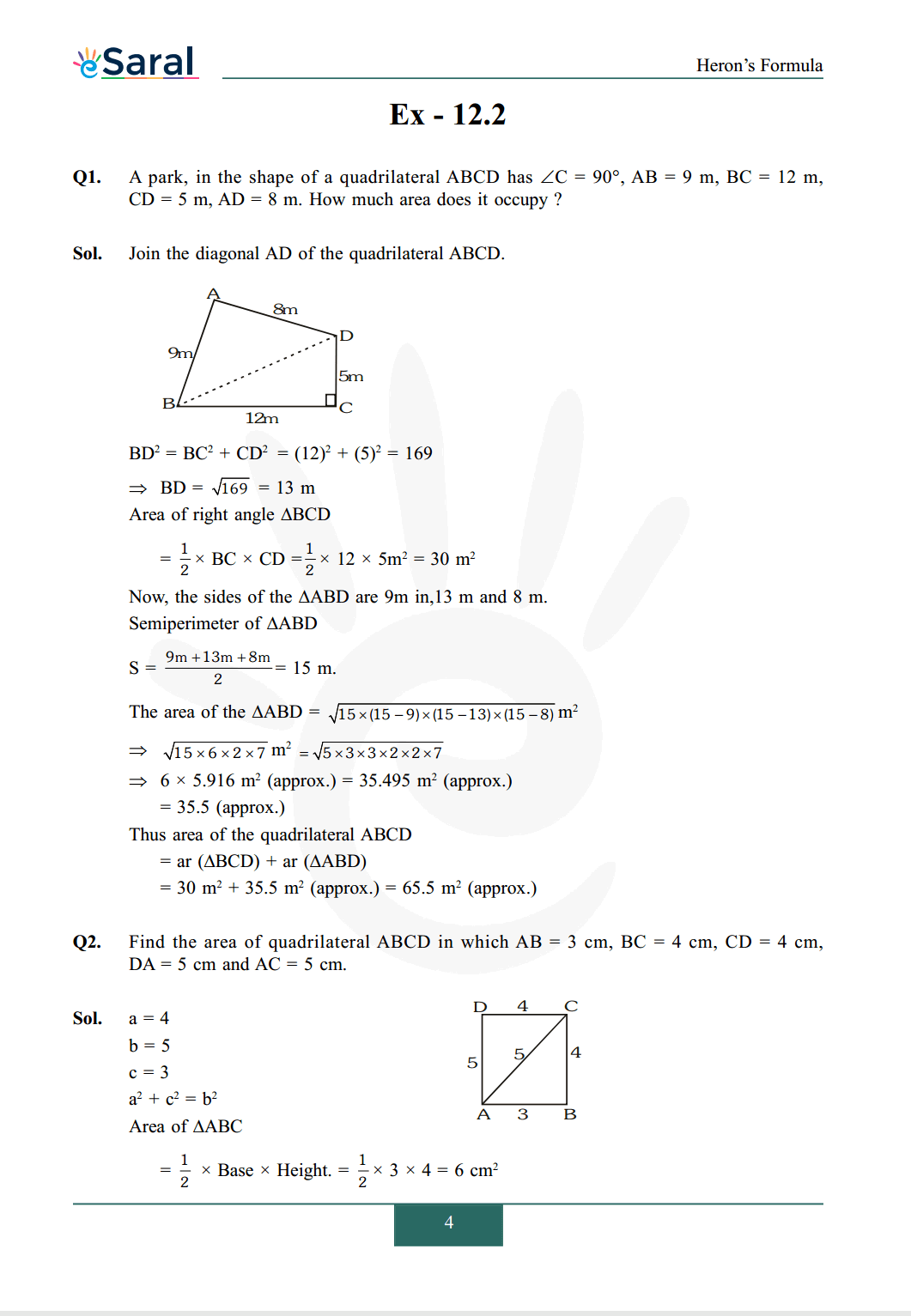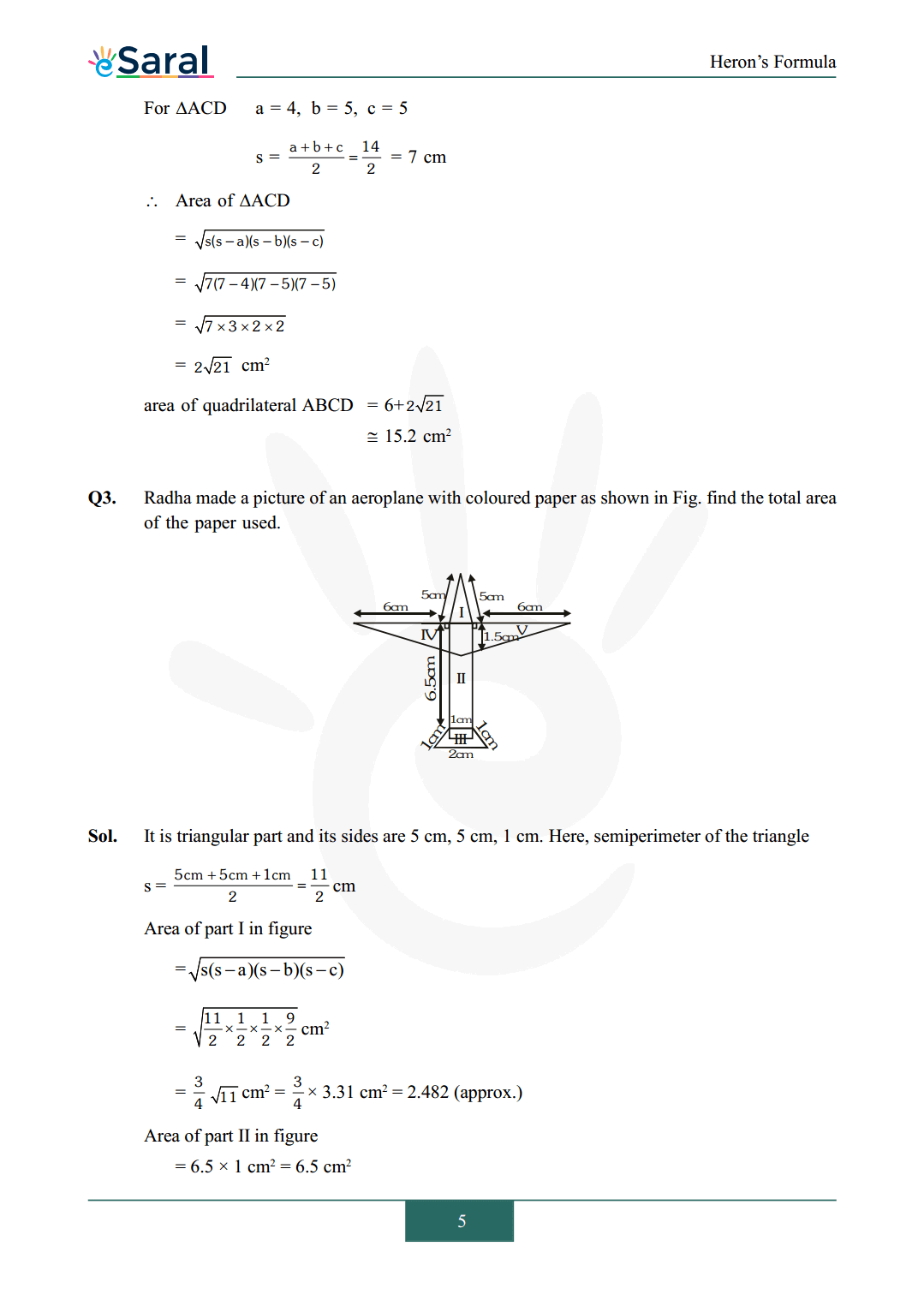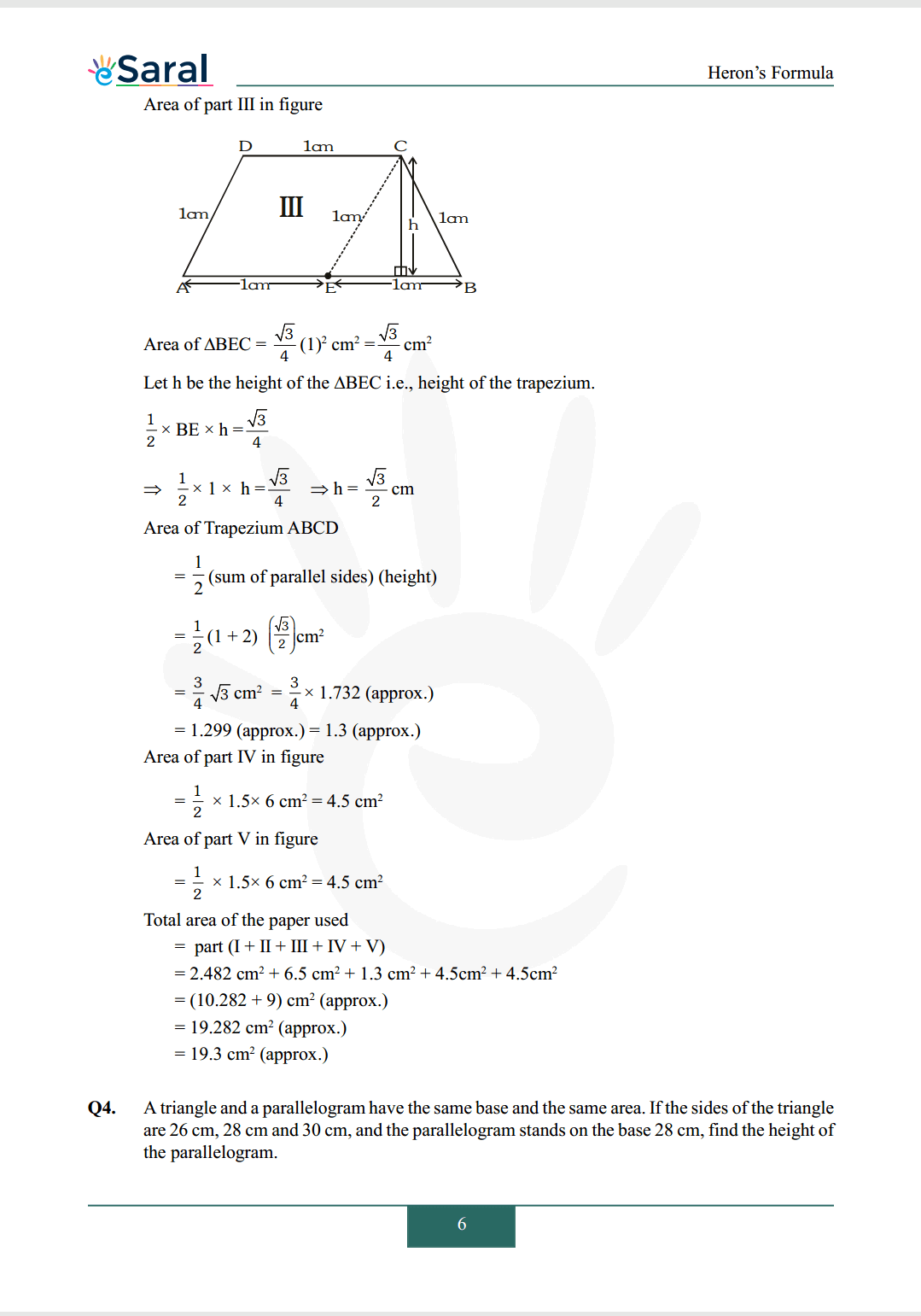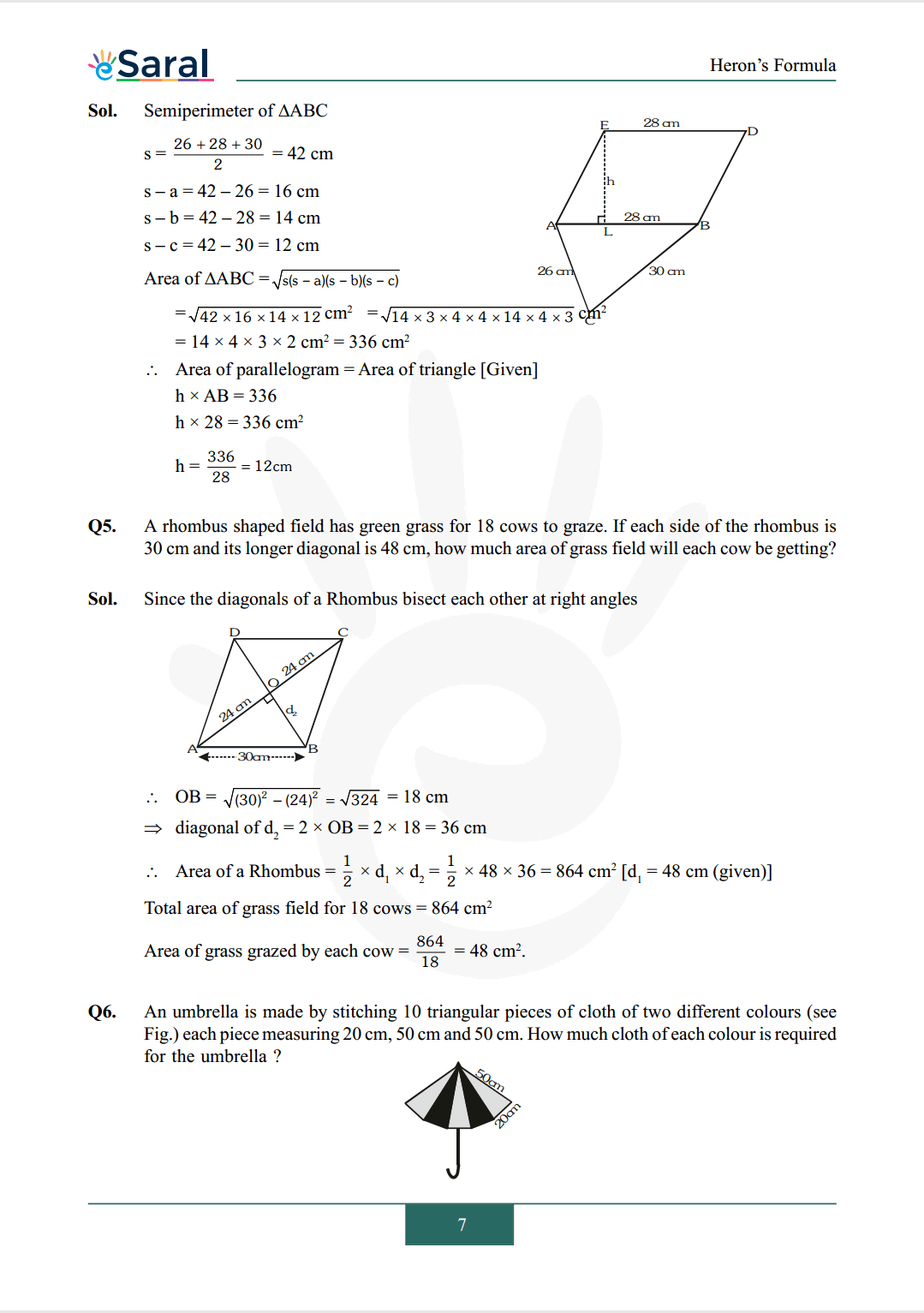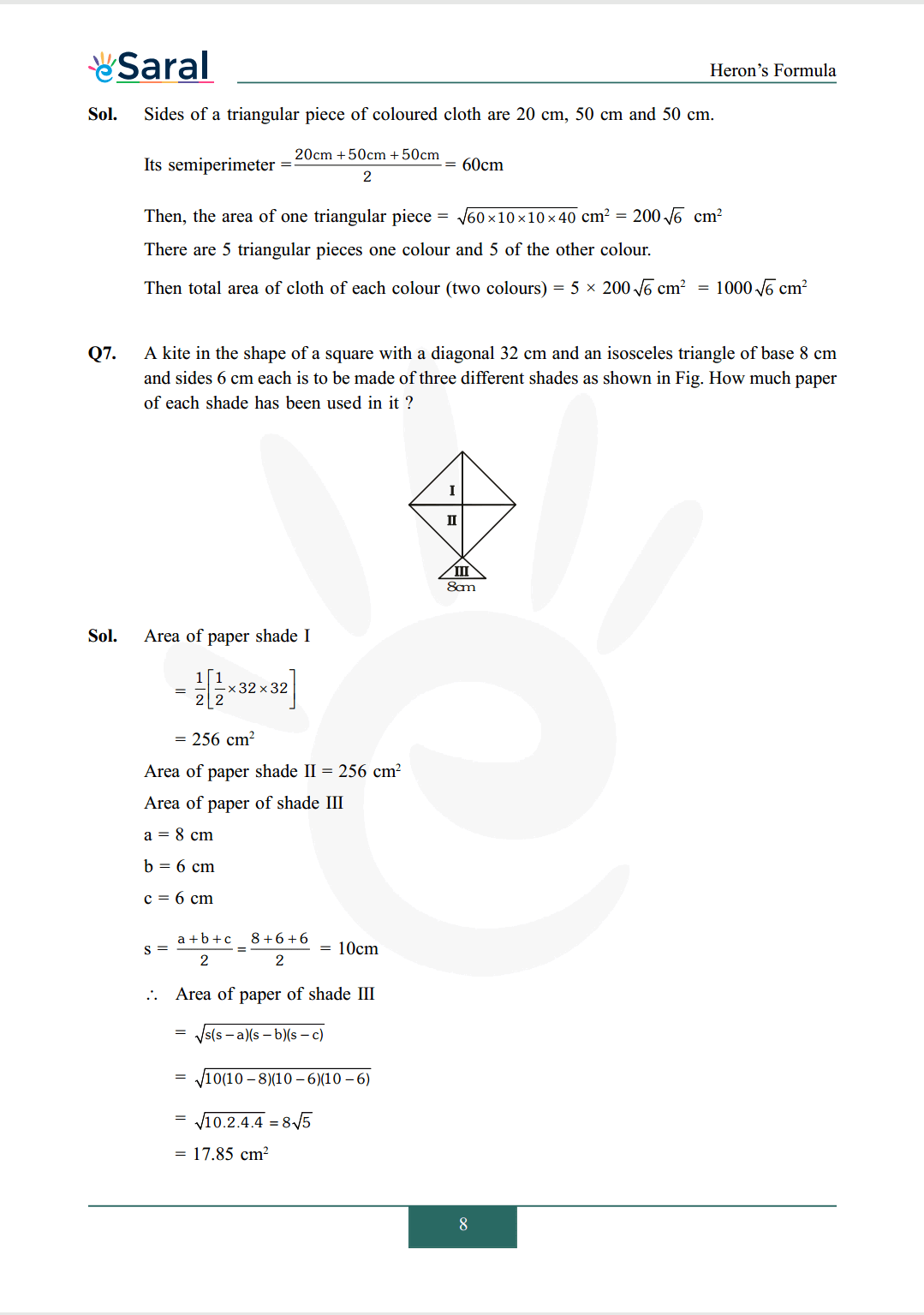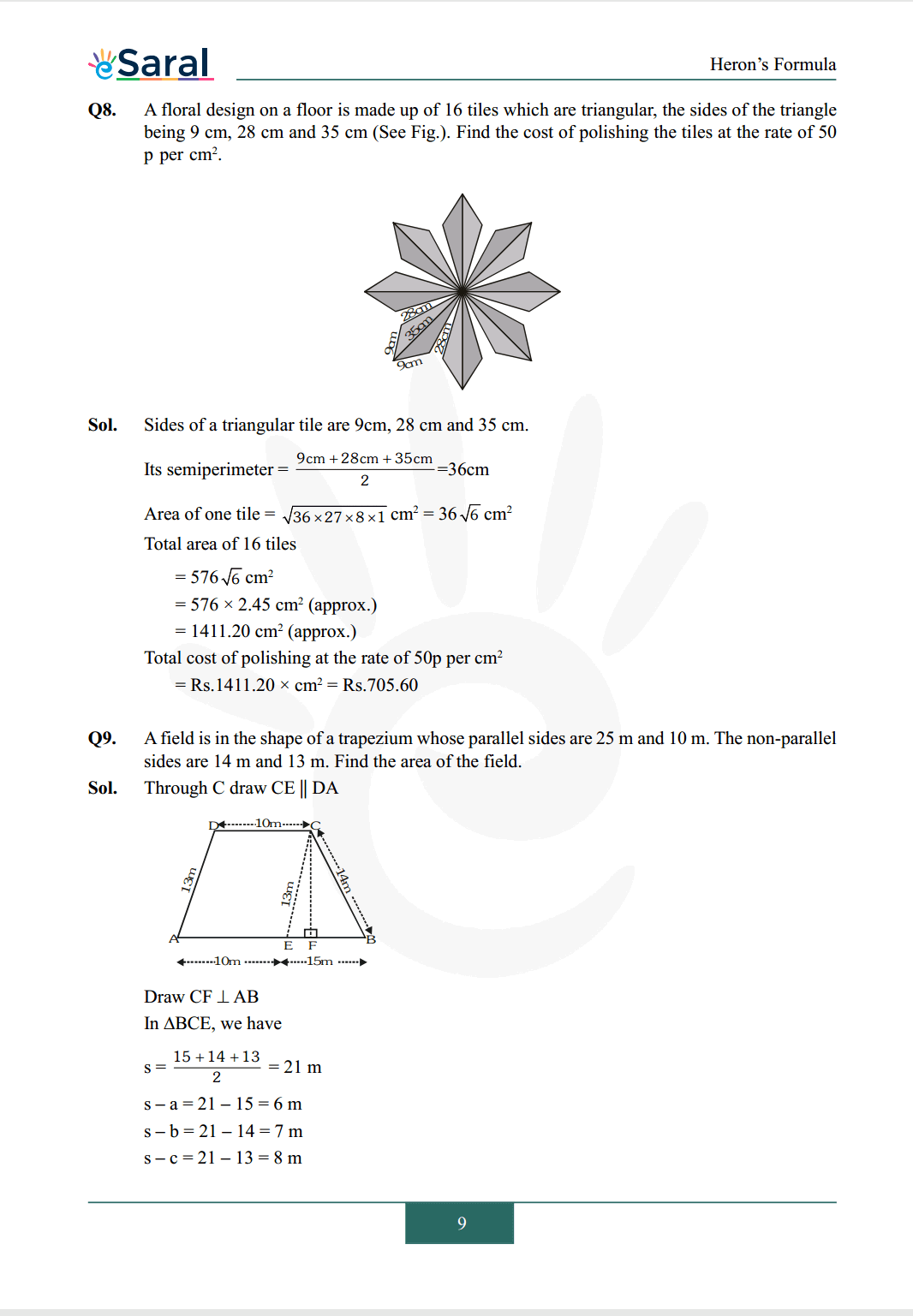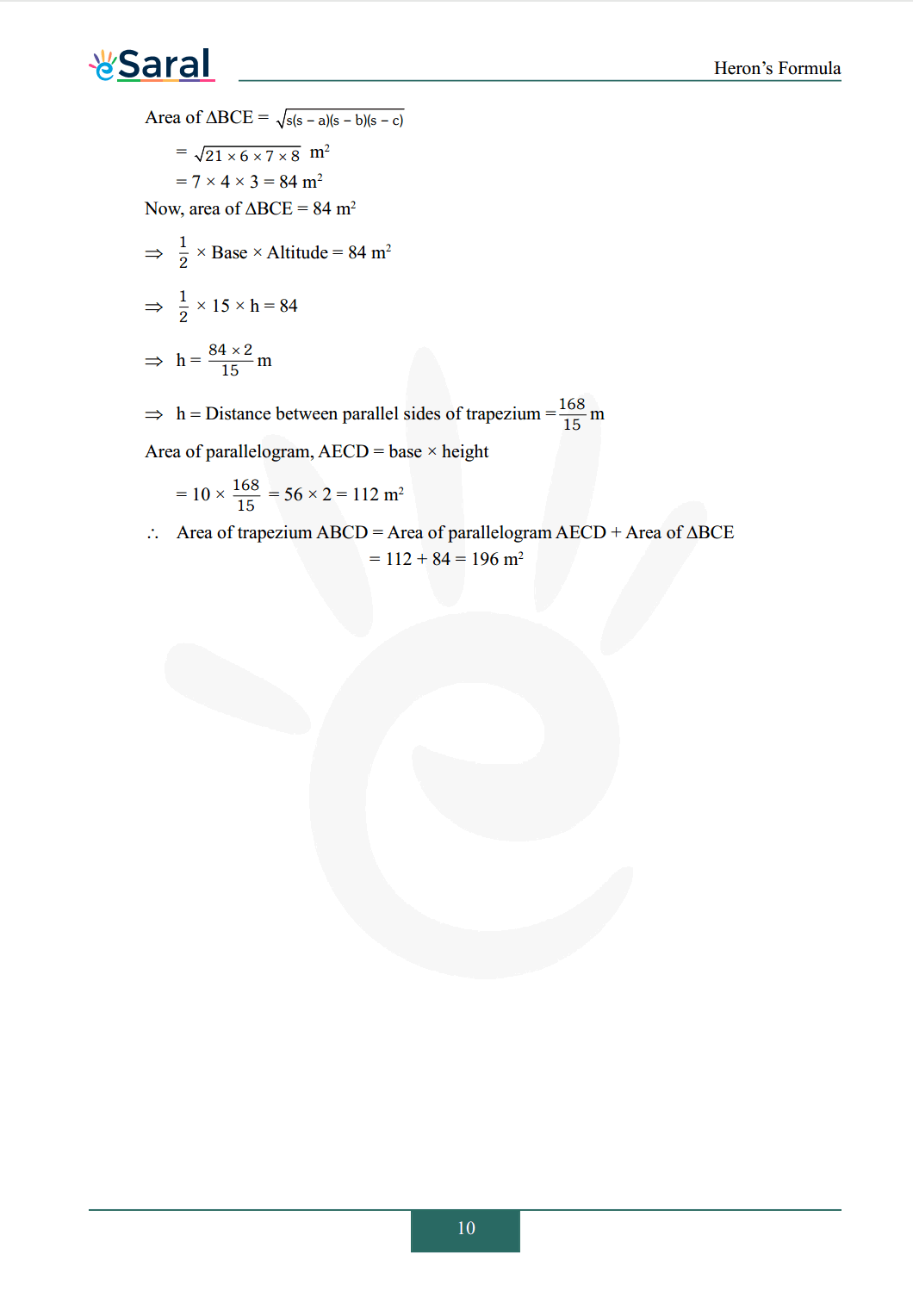#### All Questions of Chapter 12 Exercise 12.2

Once you complete the chapter 12 then you can revise Ex. 12.2 by solving following questions

Q1. A park, in the shape of a quadrilateral $\mathrm{ABCD}$ has $\angle \mathrm{C}=90^{\circ}, \mathrm{AB}=9 \mathrm{~m}, \mathrm{BC}=12 \mathrm{~m}$, $\mathrm{CD}=5 \mathrm{~m}, \mathrm{AD}=8 \mathrm{~m} .$ How much area does it occupy ?

Q2. Find the area of quadrilateral $\mathrm{ABCD}$ in which $\mathrm{AB}=3 \mathrm{~cm}, \mathrm{BC}=4 \mathrm{~cm}, \mathrm{CD}=4 \mathrm{~cm}$, $\mathrm{DA}=5 \mathrm{~cm}$ and $\mathrm{AC}=5 \mathrm{~cm}$.

Q3. Radha made a picture of an aeroplane with coloured paper as shown in Fig. find the total area of the paper used.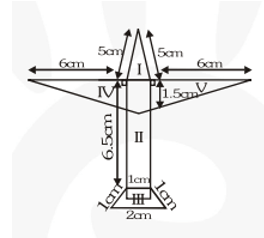Q4. A triangle and a parallelogram have the same base and the same area. If the sides of the triangle are 26 cm, 28 cm and 30 cm, and the parallelogram stands on the base 28 cm, find the height of the parallelogram.

Q5. A rhombus shaped field has green grass for 18 cows to graze. If each side of the rhombus is 30 cm and its longer diagonal is 48 cm, how much area of grass field will each cow be getting?

Q6. An umbrella is made by stitching 10 triangular pieces of cloth of two different colours (see Fig.) each piece measuring 20 cm, 50 cm and 50 cm. How much cloth of each colour is required for the umbrella ?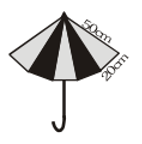Q7. A kite in the shape of a square with a diagonal 32 cm and an isosceles triangle of base 8 cm and sides 6 cm each is to be made of three different shades as shown in Fig. How much paper of each shade has been used in it ?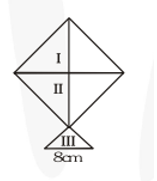Q8. A floral design on a floor is made up of 16 tiles which are triangular, the sides of the triangle being $9 \mathrm{~cm}, 28 \mathrm{~cm}$ and $35 \mathrm{~cm}$ (See Fig.). Find the cost of polishing the tiles at the rate of 50 $\mathrm{p}$ per $\mathrm{cm}^{2}$.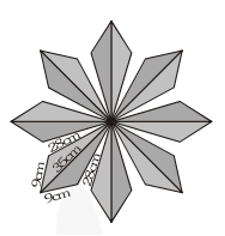Q9. A field is in the shape of a trapezium whose parallel sides are $25 \mathrm{~m}$ and $10 \mathrm{~m}$. The non-parallel sides are $14 \mathrm{~m}$ and $13 \mathrm{~m}$. Find the area of the field.

NCERT Class 9 Maths Book

NCERT Class 9 Maths Exemplar

Complete Solutions for Class 9 Maths chapter 12

Class 9 Maths Chapter 11 Exercise 11.1 Solutions

Class 9 Maths Chapter 11 Exercise 11.2 Solutions

If you have any Confusion related to NCERT Solutions for Class 9 Maths chapter 12 Exercise 12.2 then feel free to ask in the comments section down below.

To watch Free Learning Videos on Class 9 by Kota’s top Faculties Install the eSaral App